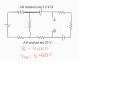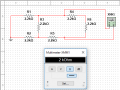# Thevenin Equivalent Circuit with multiple voltage sources.

#### ransomware

Joined Oct 9, 2017
1
In the circuit below I need to solve for Vth and Rth at points A & B.To calculate Rth, I shorted all the voltage sources and looking through points A & B, I get Rth to be 2kOhm and I verified it in multisim, however my teacher got 4.4kOhm and I am confused whether I have the right answer or not.
As for Vth, I am not sure what approach to use to solve for it's value and needed some suggestions. Thanks.#### MrAl

Joined Jun 17, 2014
8,386
Hello there,

I got 2k also and i used a different method.

But just looking at the network briefly, we can see that it can not be 4.4k. That is because the two resistors to the right in series add up to 4.4k, and there are other resistors in parallel with that so the result must be less than 4.4k. Removing R1 and R2, we are left with 4.4k in parallel with 4.4k which is 2.2k, so it must be less than 2.2k also.

#### WBahn

Joined Mar 31, 2012
26,398
One thing your teacher appears to need to do (but it might not be the best idea to suggest it too directly) is to ask if their answer makes sense.

You have (as noted by MrAl) R4+R6 = 4.4 kΩ in parallel with something else. Therefore the total resistance MUST be strictly less than 4.4 kΩ.

Looking to the left, we have R1+R2 in parallel with R3, so that branch must be less than R3 (or less than 2.2 kΩ), so the left branch, when added to R5) must be less than 4.4 kΩ, making the total strictly less than 2.2 kΩ.